# Power

Power

Power of a body is defined as the rate at which the body can do the work.

Average power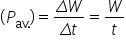Instantaneous power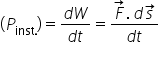[As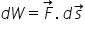]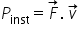i.e. power is equal to the scalar product of force with velocity.

Important Points

(1) Dimension :       [P]=[F][v]=[MLT-2][LT-1]

[P]=[ML2 T-3]

(2) Units : Watt or Joule/sec [S.I.]

Erg/sec [C.G.S.]

Practical units :  Kilowatt (kW), Mega watt (MW) and Horse power (hp)

Relations between different units : 1 watt= 1 Joule/sec = 107 erg/sec

1hp=746 Watt

1MW=106 Watt

1kW= 103 Watt

(3) If work done by the two bodies is same then power is inversely proportional to time

i.e. the body which perform the given work in lesser time possess more power and vice-versa.

(4) As power = work/time, any unit of power multiplied by a unit of time gives unit of work (or energy) and not power, i.e. Kilowatt-hour or watt-day are units of work or energy.

1kWh=3.6 * 106 Joule

(5) The slope of work time curve gives the instantaneous power. As P = dW/dt = tanθ

(6) Area under power time curve gives the work done as P=dW/dt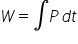W = Area under P-t curve

Related Keywords